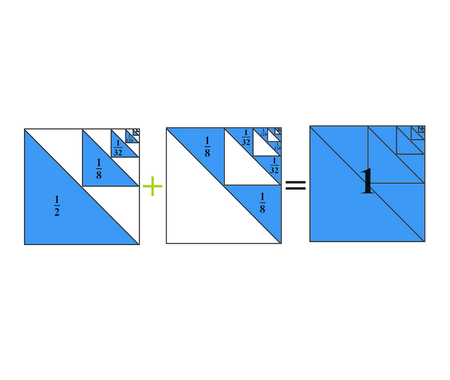## Proof without words: $$\frac{1}{2}+\frac{1}{2^{2}}+\frac{1}{2^{3}}+\cdots=1$$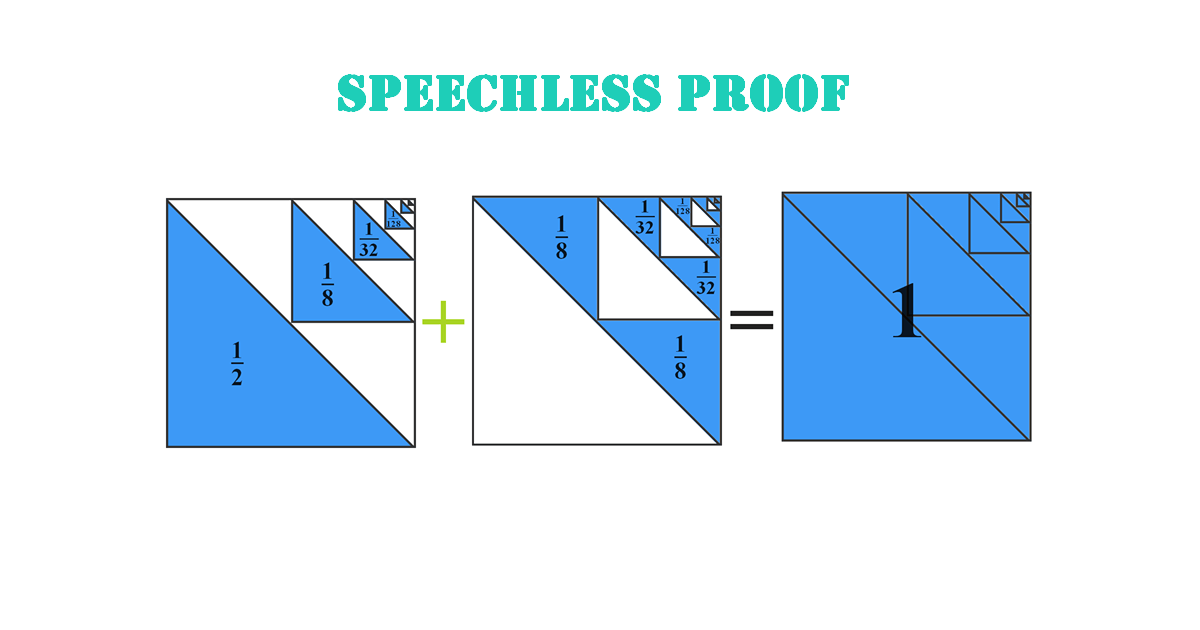### Explanation

We can extract from the next figure the following infinite sum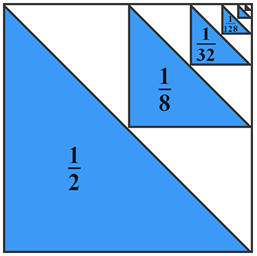$\frac{1}{2}+\frac{1}{8}+\frac{1}{32}+\frac{1}{128}+\cdots$
The same thing for the next figure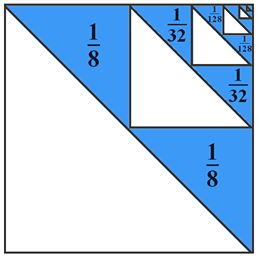$\frac{2}{8}+\frac{2}{32}+\frac{2}{128}+\frac{2}{512}+\cdots$
Now, let’s focus on the next figure to find out the result we get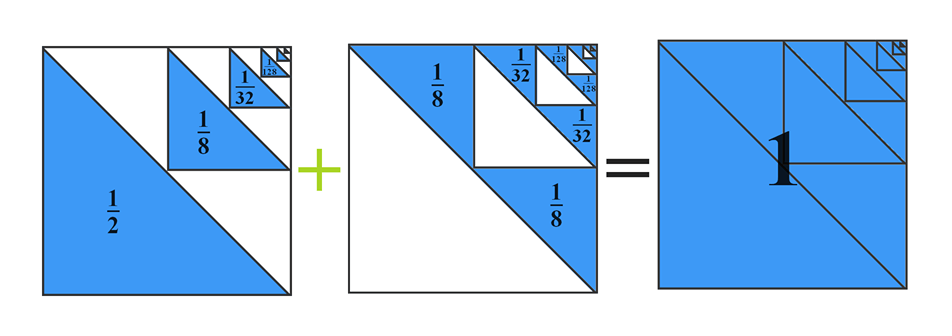\begin{aligned} &\left(\frac{1}{2}+\frac{1}{8}+\frac{1}{32}+\frac{1}{128}+\cdots\right)+\left(\frac{2}{8}+\frac{2}{32}+\frac{2}{128}+\frac{2}{512}+\cdots\right)=1 \\ &\left(\frac{1}{2}+\frac{1}{8}+\frac{1}{32}+\frac{1}{128}+\cdots\right)+\left(\frac{1}{4}+\frac{1}{16}+\frac{1}{64}+\frac{1}{256}+\cdots\right)=1 \\ &\frac{1}{2}+\frac{1}{4}+\frac{1}{8}+\frac{1}{16}+\frac{1}{32}+\frac{1}{64}+\frac{1}{128}+\frac{1}{256}+\cdots=1 \\ &\frac{1}{2}+\frac{1}{2^{2}}+\frac{1}{2^{3}}+\frac{1}{2^{4}}+\frac{1}{2^{5}}+\frac{1}{2^{6}}+\frac{1}{2^{7}}+\frac{1}{2^{8}}+\cdots=1 \end{aligned}

### Related Topics

Find the volume of the square pyramid as a function of $$a$$ and $$H$$ by slicing method.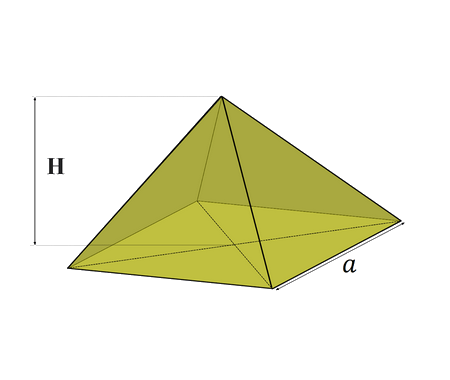Prove that $\lim_{x \rightarrow 0}\frac{\sin x}{x}=1$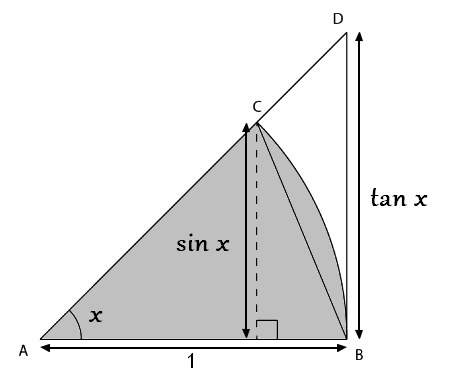Prove that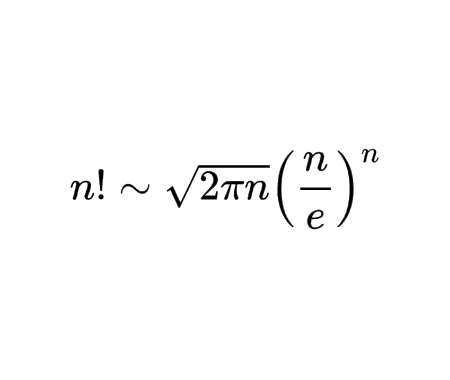Calculate the half derivative of $$x$$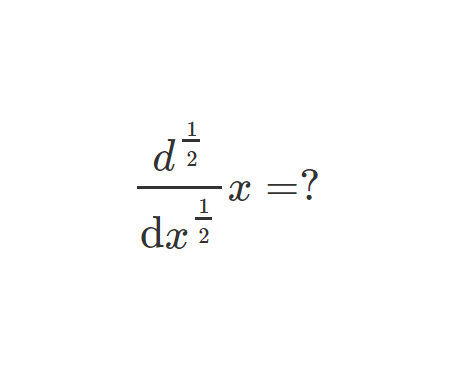Prove Wallis Product Using Integration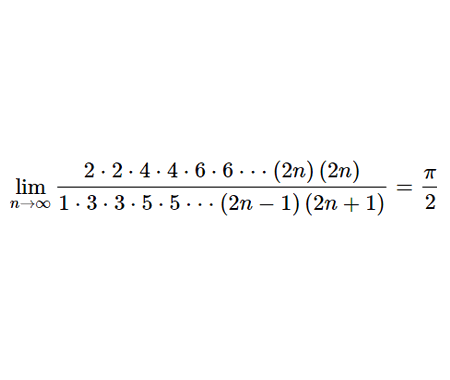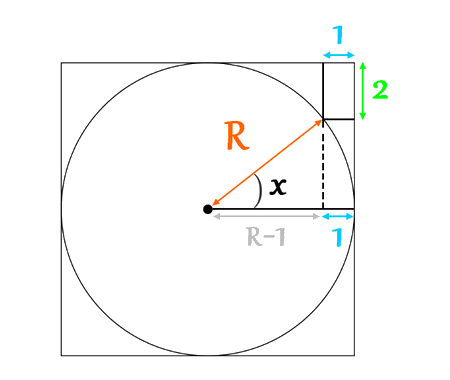Calculate the volume of Torus using cylindrical shells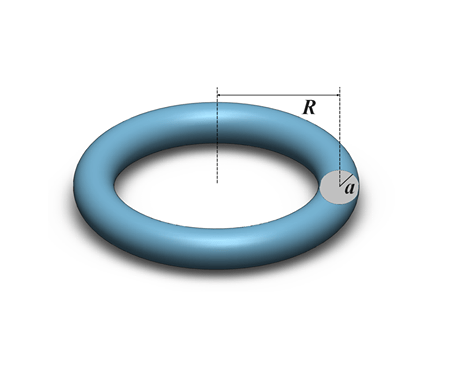Find the derivative of exponential $$x$$ from first principles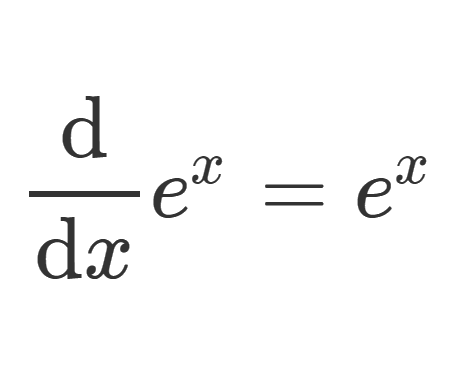Calculate the sum of areas of the three squares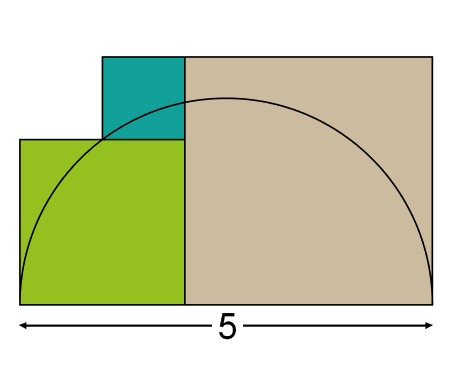Find the equation of the curve formed by a cable suspended between two points at the same height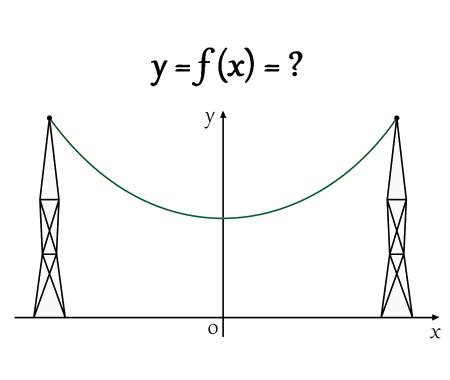Solve the equation for real values of $$x$$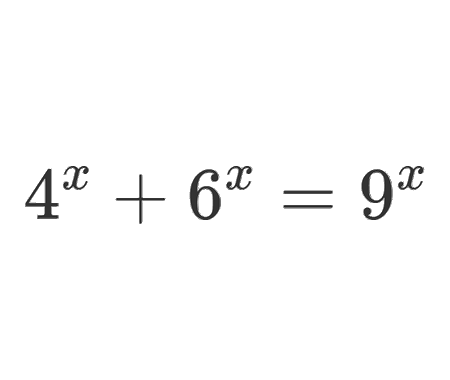Solve the equation for $$x\epsilon\mathbb{R}$$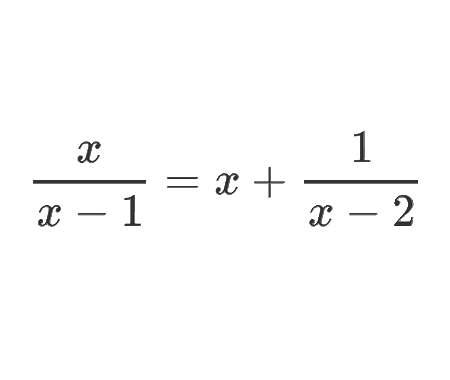Determine the angle $$x$$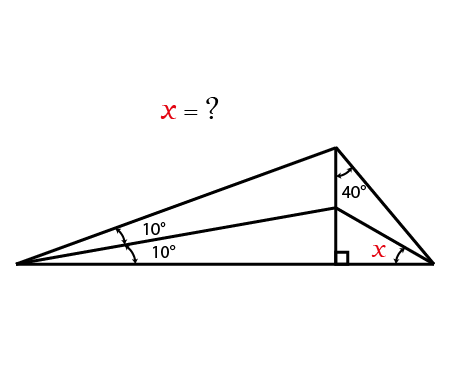Calculate the following limit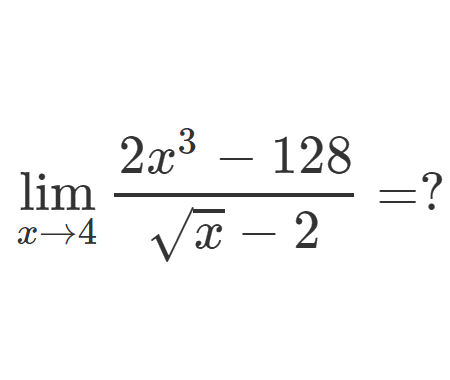Calculate the following limit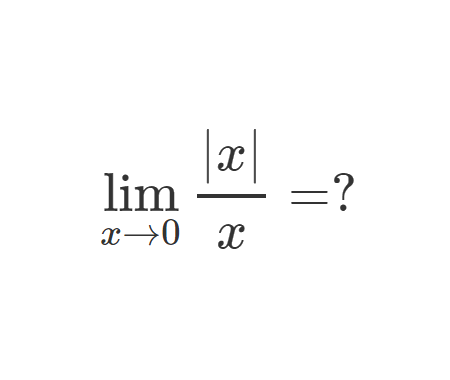Calculate the integral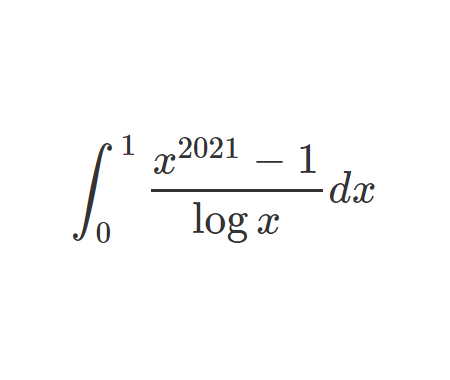Challenging problem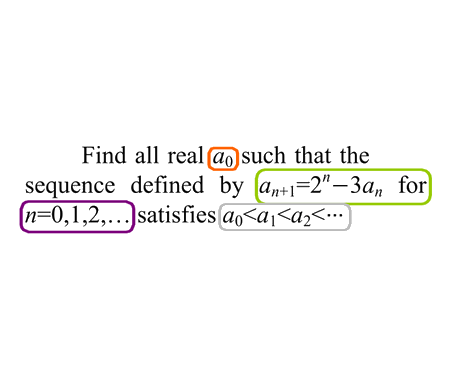Speechless proof